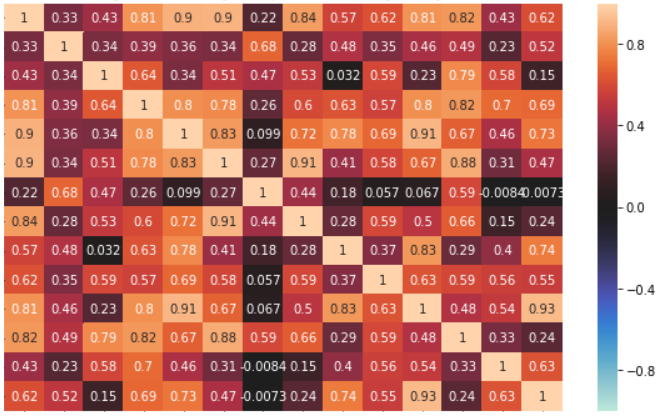# Why the Correlation Coefficient r ranges between -1 and +1.?Image by Author – Correlation Matrix

Correlation Coefficient is a statistical measure of the strength of the relationship between the relative movements of two variables.

We know that the equation of Sum of Squared Errors is as below.

If there is a perfect Linear Regression exists between 2 variables x & y, then the line of regression runs through all the points and so there will be no error.

This is the maximum value of r² which is 1.

The maximum SSE occurs only when there is no correlation. i.e minimum correlation.

This means that r² is 0 which is the minimum value of r².

If the gradient (i.e. Slope) of line of regression is -ve, then Sxy <0. So r² < 0.

If it is +ve, then Sxy > 0. So r > 0.

Hence -1≤ r ≤+1

0